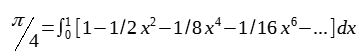top of page
Search

# Speciality of unconventionality

A week ago, on 22nd of July (22/7) was pi approximation day and this post, is aimed towards looking at how brilliant minds progressed towards earning bragging rights. In the early days, the methods and ideas to calculate the value of pi was meticulously slow and in a sense exhausting. However, as we have talked a lot about one guy on this blog, he makes his way through in this post too. Isaac Newton as usual with his class act of coming up with intuitive ideas, outwitted his brethren.This is Pascal's triangle and the basic idea behind it is, if you look at the coefficients of x, x squared and so on. They are actually just the numbers in pascal's triangle. The power of (1+x) corresponds to the row in pascal's triangle. It's something that has been known for a considerable number of years. Whenever you have a row, you just add the two neighbors and that gives you the value of the row below it, as a consequence you can probably calculate the coefficients to the power ten, instead of doing all the algebra. Over the years, people unraveled a general formula for the numbers in Pascal's triangle and that my friends is, the binomial theorem.Binomial, as the name suggests it gives us an expansion for any two terms and theorem because you could put in values in place of 'x' and show that the coefficients match the values in Pascal's triangle. This is where Newton demonstrated his brilliance for probably the ten time now, from all the endeavours that he had already demonstrated. The conventional requisites of the theorem suggests that you plug in only positives integers of 'n'. But as usual breakthroughs most likely happen when you try to go out and commit to unconventional tasks. And so, Newton plugged in all sorts of values, from negatives to fractions.

He starts with n equals a negative one (it gives 1/(1+x) on the left-hand side), what happens? if you plug a negative one in the above equations, the signs of the coefficients alternate or shift between positive and negative respectively, on the right-hand side to infinity. As a consequence, if you don't have a positive integer, the binomial theorem just gives an infinite series. And you could check this for yourself by plugging in a positive integer, the series at the value of '(n-n)' becomes zero, as a result leaving with a finite value in the case of a positive integer.

What Newton did (you could do this too and gain satisfaction towards the result) was basically multiply both the sides by (1+x), which essentially cancelled out most of the terms on the left-hand side, and left the value '1'(right hand side - 1/(1+x) * (1+x) leaving '1' as the end result, lhs=rhs). That's how Newton figured it out that it's reasonable that the formula works with his substitutions. As a result, implying that there is more to the pascal's triangle than just the positive part. Anyway, he doesn't stop there, he goes ahead and plugs in a half. Leaving us with an infinite series of the sort,Essentially the idea behind this is that, can be thought if like layers in the triangle which add up to give more layer or a say a plane. At this point you could basically plug in anything into the theorem and calculate the values of various square roots in this case, to a greater precision. Since, Newton wanted to calculate the value of pi, he was interested in the value where n equalled half, why?

The equation of a unit circle is,

x² + y² = 1

=> y= √(1-x²)

This is essentially the same equation as above by substituting '-x²' in place of 'x'.He represents 'y' in two ways and basically uses calculus, he integrates the above equation from 0 to 1, since its a unit circle and he knows that the area of a unit circle is just pi because the radius is equal to one. And since he is integrating the above equation from 0 to 1, he is only looking for a quarter of the area of the circle that is, pi over four.He just takes the four to the other side and substitutes the limits to calculate the value of pi. Newton doesn't stop there, instead of integrating 0 to 1, he integrates from 0 to 1/2. The idea behind this is, when you have an infinite series, you want the values to keep decreasing as quickly, which has a sole purpose of calculating things with utmost precision. So, Newton comes to comprehend that when he replaces the limit ti 1/2, he reduces each term by an addition factor of x squared. Now, the area that he'll be calculating with the new limit is, the portion AOB plus BOC, Now BOC is a thirty-degree sector of the circle which has an area of pi over twelve and AOC is a right triangle with a base of half and height of root three on two, from the unit circle and hence you get the left-hand side given below.After some tedious rearrangement...You end up with,Before Isaac Newton people inscribed unit circles into polygons and calculated the side lengths, which made the process very exhausting and none looked at what Newton showed in the coming decades.

Moral of the story : Always strive for the most unconventional methods while trying out new things, even if it demands more efforts, because the results are going to be worth that extra effort. And so, conventionally it is the most unconventional ideas that most likely dictate our understanding of various aspects of life.